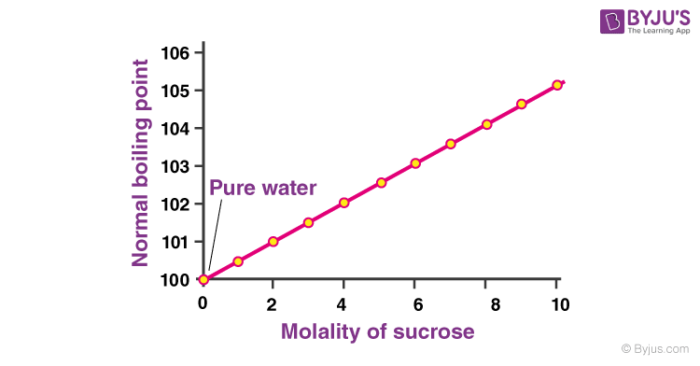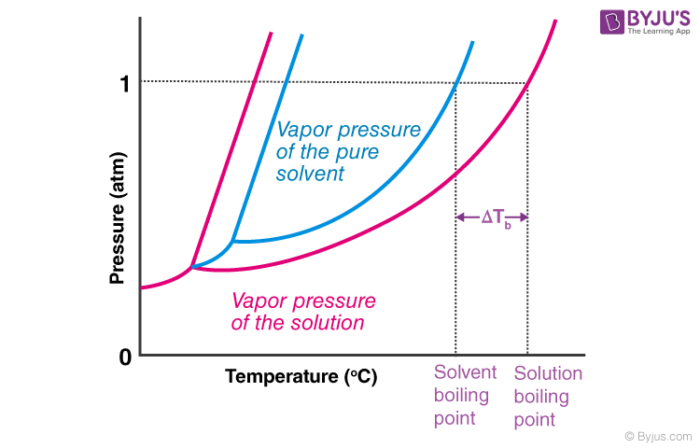# Boiling Point Elevation

## What is Boiling Point Elevation?

Boiling point elevation refers to the increase in the boiling point of a solvent upon the addition of a solute. When a non-volatile solute is added to a solvent, the resulting solution has a higher boiling point than that of the pure solvent. For example, the boiling point of a solution of sodium chloride (salt) and water is greater than that of pure water.

Boiling point elevation is a colligative property of matter, i.e. it is dependent on the solute-to-solvent ratio but not on the solute’s identity. This implies that the elevation in the boiling point of a solution depends on the amount of solute added to it. The greater the concentration of solute in the solution, the greater the boiling point elevation.A graph detailing the elevation in the boiling point of water upon the addition of sucrose is provided above. At 1atm of pressure, pure water boils at 100oC. However, a 10 molal solution of sucrose in water boils at approximately 105oC.

## Why Does Boiling Point Elevation Occur?

The boiling point of a liquid is the temperature at which its vapour pressure is equal to the pressure of its surrounding environment. Non-volatile substances do not readily undergo evaporation and have very low vapour pressures (assumed to be zero). When a non-volatile solute is added to a solvent, the vapour pressure of the resulting solution is lower than that of the pure solvent.

Therefore, a greater amount of heat must be supplied to the solution for it to boil. This increase in the boiling point of the solution is the boiling point elevation. An increase in the concentration of added solute is accompanied by a further decrease in the vapour pressure of the solution and further elevation in the boiling point of the solution.

A pressure v/s temperature graph detailing the boiling point elevation of a solution is provided below.Here, ΔTb represents the elevation in the boiling point of the solution. From the graph, it can be observed that –

1. The freezing point of the solution is lower than that of the pure solvent (freezing point depression).
2. The boiling point of the solution is higher than that of the pure solvent.

Note: The boiling point of a liquid is also dependent on the pressure of its surroundings (which is why water boils at temperatures lower than 100oC at high altitudes, where the surrounding pressure is low).

## Boiling Point Elevation Formula

The boiling point of a solution containing a non-volatile solute can be expressed as follows:

Boiling point of solution = boiling point of pure solvent + boiling point elevation (ΔTb)

The elevation in boiling point (ΔTb) is proportional to the concentration of the solute in the solution. It can be calculated via the following equation.

ΔTb = i×Kb×m

Where,

• i is the Van’t Hoff factor
• Kb is the ebullioscopic constant
• m is the molality of the solute

It is important to note that this formula becomes less precise when the concentration of the solute is very high. Also, this formula doesn’t hold true for volatile solvents.

The ebullioscopic constant (Kb) is often expressed in terms of oC/molal or oC.kg.mol-1. The values of Kb for some common solvents are tabulated below.

 Solvent Kb value (in oC.kg.mol-1) Water 0.512 Phenol 3.04 Acetic Acid 3.07 Chloroform 3.63 Benzene 2.53

The degree of dissociation of the solute and the molar mass of the solute can be calculated with the help of the boiling point elevation formula.

## Solved Examples

### Example 1

Calculate the boiling point of a 3.5% solution (by weight) of sodium chloride in water.

1 kg of the given solution contains 0.035kg of NaCl and 0.965kg of H2O. Since the molar mass of NaCl is 58.5, the number of moles of NaCl in 1 kg of the solution is:

(35g)/(58.5g.mol-1) = 0.598 moles

The molality of NaCl in 1kg of the solution can be calculated as:

m = (0.598mol)/(0.965 kg) = 0.619 molal

The boiling point elevation constant of water is 0.512 oC.kg/molal. Since NaCl dissociates into 2 ions, the Van’t Hoff factor for this compound is 2. Therefore, the boiling point elevation (ΔTb) can be calculated as follows:

ΔTb = 2×(0.52oC/molal)×(0.619 molal) = 0.643oC

Boiling point of the solution = boiling point of pure solvent + boiling point elevation

= 100oC + 0.643oC = 100.643oC

Therefore, the boiling point of the 3.5% NaCl solution is 100.643oC.

### Example 2

10 grams of a non-volatile and non-dissociating solute is dissolved in 200 grams of benzene. The resulting solution boils at a temperature of 81.2oC. Find the molar mass of the solute.

Let x = number of moles of solute. The boiling point of pure benzene is 80.1oC and it’s ebullioscopic constant is 2.53oC/molal. From the boiling point elevation formula, the following relationship can be obtained:

(81.2oC – 80.1oC) = (1)×(2.53oC.kg.mol-1)(x/0.2 kg)

x = (1.1oC×0.2kg)/(2.53oC.kg.mol-1)

x = 0.0869 moles

Since 0.0869 moles of the solute has a mass of 10 grams, 1 mole of the solute will have a mass of 10/0.0869 grams, which is equal to 115.07 grams. Therefore, the molar mass of the solute is 115.07 grams per mole.